### AP Physics 1 Week 3 (June 25 - July 01, 2017)

Part 1:  Algebra (Rearrange)

1.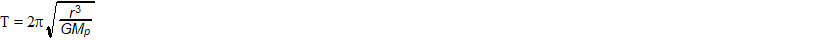solve for Mp

2.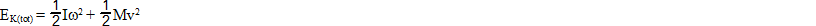solve for w

3.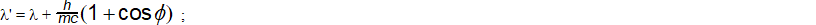solve for c

4.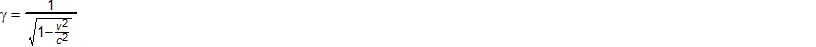solve for c

5.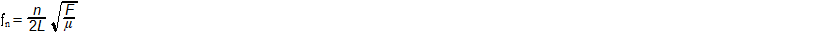solve for m

Part 2:  Algebra (Plug and Chug)

6.r = 6.371 x 106, G = 6.67 x 10-11, Mp = 1.989 x 1030, solve for T

7.I = 2.67, w = 2.95, M 0.546, v = 6.78, solve for EK(tot)

8.solve for l' when,  l = 3.89 x 10-7h = 6.626 x 10-34, m = 9.11 x 10-31, c = 3 x 108f is 37

9.solve for when v = 2.95 x 108, c = 3 x 108

10.solve for fn when n = 4, L = 16, F = 15, m = 6.38

Part 3: Geometry/Trigonometry
(For Physics, make sure your calculator is set in degrees)
Frequently in Physics and Astronomy we must represent angular measurements in the form of degrees, arcminutes and arcseconds, rather than decimal degrees.  Your TI-8x calculator can actually perform this function listed under catalog (above the zero).  23.569875 degrees can be converted to degrees-minutes-seconds under the catalog function as >DMS to become 23o 34' 11.55", read as 23 degrees, 34 arcminutes, 11.55 arcseconds.  This can also be done by hand, whereas, there are 60 arcminutes in 1 degree, and 60 arcseconds in 1 arc minute or 3600 arcseconds in 1 degree.

Use your calculator converter under catalog to convert the following decimal degrees to degrees-minutes-seconds

11.  18.563248o

12.  125.32657o

13.  168.523658o

14.  255.325684o

15.  53.56987o

Part 4:  Word Problems

speed = (distance / time) or speed x time = distance,  speed is a "rate"

Special Note:  WKP and WKS is the first letter of the authors last name of the textbook this problem was taken.

Need help:   check out:

http://www.onlinemathlearning.com/distance-problems.html

16.  At 10:00 am two planes leave an airport.  If the northbound plane flies at 280 mph

(miles per hour), and the southbound plane flies at 320 mph, at what time will

they be 1000 miles apart? (DBW, p66, #16)

17.  A boy leaves on a bicycle trip at a speed of 8 mph.  One hour after departure, his

father, realizing that his son forgot some camping gear, sets out by car.  How

fast must the father drive to overtake the boy in 15 minutes?  (Assume constant

velocity, neglecting any time for acceleration/deceleration) (DBW, p66, #17)

18.  Davis made a car trip in 3 hours, while the return trip took 3.5 hours.  If his speed

going was 5mph more than his speed returning, how many miles was the total or             round trip? (DBW, p67, #20)

19.  If Jane were able to increase her cycling speed by 4mph, she would be able to

cover in 2 hours a distance that now takes her 3 hours.  What is the best speed

she achieves at present? (DBW, p67, #28)

20.  A "local" train leaves a station and runs at an average of 35 mph .  An hour and a

half later, an "express" train leaves the station and travels at an average speed

of 56 mph on a parallel track.  How many hours after it starts will the "express"

train overtake the "local" train? (DBW p75, #44)